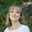# Pandas cheat sheet: Top 35 commands and operations

Jun 30, 2020 - 7 min readAmanda Fawcett## Beginner Pandas Series

• #### Pandas Cheat Sheet: top 35 commands and operations

Pandas is one of the most popular tools for data analysis in Python. This open-source library is the backbone of many data projects and is used for data cleaning and data manipulation.

With Pandas, you gain greater control over complex data sets. It’s an essential tool in the data analysis tool belt. If you’re not using Pandas, you’re not making the most of your data.

In this post, we’ll explore a quick guide to the 35 most essential operations and commands that any Pandas user needs to know.

Let’s get right to the answers.

## Pandas import convention

import pandas as pd


Pandas is now accessible with the acronym pd. You can also install Pandas using the built-in Python tool pip and run the following command.

\$ pip install pandas


## Create and name a Series

Create one-dimensional array to hold any data type. Invoke the pd.Series() method and then pass a list of values. Pandas will default count index from 0.

series1 = pd.Series([1,2,3,4]), index=['a', 'b', 'c', 'd'])


Set the Series name

srs.name = "Insert name"


Set index name.

srs.index.name = "Index name"


## Create a DataFrame

Create a two-dimensional data structure with columns. Create and print a df.

df = pd.DataFrame(
{"a" : [1 ,2, 3],
"b" : [7, 8, 9],
"c" : [10, 11, 12]},        index = [1, 2, 3])


## Specify values in DataFrame columns

Specify how you want to organize your DataFrame by columns.

df = pd.DataFrame(
[[1, 2, 3],
[4, 6, 8],
[10, 11, 12]],
index=[1, 2, 3],
columns=['a', 'b', 'c'])


## Read and Write to CSV file

Open the CSV file, copy the data, paste it in our Notepad, and save it in the same directory that houses your Python scripts. Use read_csv function build into Pandas and index it the way we want.

import pandas as pd



## Read and write to Excel file

Call the read_excel function to access an Excel file. Pass the name of the Excel file as an argument.

pd.read_excel('file.xlsx')

df.to_excel('dir/myDataFrame.xlsx',  sheet_name='Sheet2')


## Read and write to SQL Query

from sqlalchemy import create_engine
engine = create_engine('sqlite:///:memory:')


(read_sql() is a convenience wrapper around read_sql_table() and read_sql_query())

df.to_sql('myDf', engine)


## Get the first element of a Series

Since Pandas indexes at 0, call the first element with ser.

import pandas as pd

# get the first element
ser


## Get the first 5 elements of a Series

Use ser[:n] to get the first $n$ elements of a Series.

import pandas as pd

ser[:5]


## Get the last 5 elements in a Series

Use ser[-n:] to get the last $n$ elements of a Series.

import pandas as pd

ser[-5:]


## Select a single value position

df.iloc[,] 'Name'
df.iat(,) 'Name'


## Select a single value by label

df.loc[, ['Label']] 'Name'
df.at(, ['Label']) 'Name'


## Access a DataFrame with a boolean index

In boolean indexing, we filter data with a boolean vector.

import pandas as pd

# dictionary of lists
dict = {'name':["name1", "name2", "name3", "name4"],
'degree': ["degree1", "degree2", "degree3", "degree4"],
'score':[1, 2, 3, 4]}

df = pd.DataFrame(dict, index = [True, False, True, False])

print(df)


## Drop values from rows

s.drop(['a', 'c'])


## Drop values from columns

df.drop('Value', axis=1)


## Create a new column in a DataFrame

df['New Column'] = 0


### Keep the learning going.

Learn Pandas and Data Analysis without scrubbing through videos or documentation. Educative’s text-based courses are easy to skim and feature live coding environments, making learning quick and efficient.

Predictive Data Analysis with Python

## Rename columns in a DataFrame

df.columns = ['Column 1', 'Column 2', 'Column 3']


## Sort Series by labels along an axis

Sort Series by index labels and returns a new Series sorted by the label if inplace argument is False, otherwise it updates the original series and returns None.

Series.sort_index(self, axis=0, level=None, ascending=True, inplace=False, kind='quicksort', na_position='last', sort_remaining=True)


## Sort values along an axis (ascending order)

df.sort_values(by='Values')

# descending order
df.sort_values(ascending = False)


## Adding ranks to particular entries

Specify how you want to rank a column and add ranks.

df.rank()


## Retrieve rows and columns description

df.shape


## Describe columns of DataFrame

df.columns


## Retrieve index description

df.index


## Get information on DataFrame

df.info()


## Retrieve number of non-NA values

df.count()


## Get sum of values

df.sum()

# cumulative sum

df.cumsum()


## Subtract/Add 2 from all values

s.sub(2)



## Multiply/Divide all values by 2

s.mul(2)

s.div(2)


## Find min/max values of a DataFrame

df.min()

df.max()


## Get min/max index values

df.idxmin()

df.idxmax()


## Get median or mean of values

df.mean()

df.median()


## Describe a summary of data statistics

df.describe()


## Apply a function to a dataset

f = # write function here
df.apply(f)

# apply a function by an element

f = # write function here
df.applymap(f)


## Merge two DataFrames

pd.merge(df1, df2, on='subject_id')


## Combine DataFrames across columns or rows: concatenation

print(pd.concat([df1, df2]))## Wrapping up and resources

Now that you’re armed with the common operations and commands in Python, you can put them into practice. After all, working with real datasets is the best way to master Python and become a data analyst! There’s still a lot more to learn that we didn’t cover today such as:

To get started with these essential tools of the trade, check out Educative’s course Predictive Data Analysis for Python. You’ll get hands-on practice with industry-standard examples and become fluent in the data analysis.